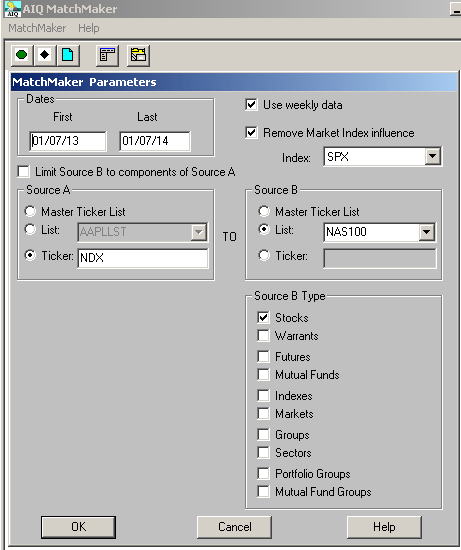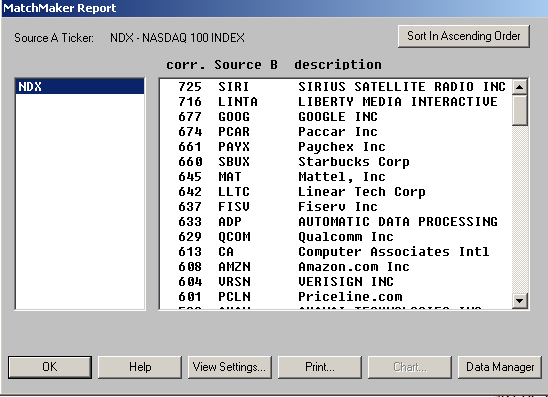# Timing The Market With Pairs Logic

The AIQ code based on Perry Kaufman’s article in March 2014 Stocks & Commodities, “Timing The Market With Pairs Logic,” is provided at www.TradersEdgeSystems.com/traderstips.htm.

The code I am providing will backtest only the long trading and will not test the hedging portion of the system. For live trading, I provided a manual input for the total value of the open positions, which would have to be computed separately and then entered daily as an input before the daily report is run once the hedge rule becomes true.

```!TIMING THE MARKET WITH PAIRS
!Author: Perry Kaufman, TASC March 2014
!Coded by: Richard Denning 1/7/2014
!INPUTS:
stoLen is 60.
idx is "NDX".
hedgeETF is "PSQ".
eLvl is 10.
xLvl is 50.
sLoss is 0.10.
hedgeLen is 60.
hedgeRatio is 0.50.
minP is 3.
invest is 5000.
totValOpenPos is 100000. !open position value must be set manually
H is [high].
L is [low].
C is [close].
O is [open].
idxH is tickerUDF(idx,H).
idxL is tickerUDF(idx,L).
idxC is tickerUDF(idx,C).
idxO is tickerUDF(idx,O).
hedgeC is tickerUDF(hedgeETF,C).
PEP is {position entry price}.
!STRESS CODE:
rngStk is highresult(H,stoLen) - lowresult(L,stoLen).
rngIdx is highresult(idxH,stoLen) - lowresult(idxL,stoLen).
stoStk is (C - lowresult(L,stoLen)) / rngStk.
stoIdx is (idxC - lowresult(idxL,stoLen)) / rngIdx.
stoDiff is stoStk - StoIdx.
rngDiff is highresult(stoDiff,stoLen) - lowresult(stoDiff,stoLen).
stressVal is ((stoDiff - lowresult(stoDiff,stoLen)) / rngDiff) * 100.
!PAIRS SYSTEM CODE:
Buy if C > minP and countof(C > minP,4,1)=4 and stressVal <= eLvl and symbol()<>hedgeETF.
StressExit if stressVal >= xLvl.
ExitBuy if countof(C > minP,5)<>5 or C/PEP-1 < -sLoss or StressExit.
!TREND CODE:
idxTrnd is simpleavg(idxC,hedgeLen).
Hedge if idxTrnd < valresult(idxTrnd,1) and symbol()=hedgeETF .
!SIZING CODE:
chgStk is C/valresult(C,1) - 1.
chgIdx is idxC/valresult(idxC,1) - 1.
idxSMA is simpleavg(idxC,hedgeLen).
size is floor(invest / C).
hedgeSize is floor(totValOpenPos / hedgeC*hedgeRatio) .
ShowAllValues if countof(C > minP,5)=5.```

To get a correlated list of stocks that show good correlation to the index of choice (I used the NDX), AIQ has a matchmaker module that will quickly generate a list of stocks that show significant correlation to an index. In Figure 7, I show the matchmaker setup I used to quickly get a list of stocks in the NASDAQ 100 that were highly correlated to the NDX. In Figure 8, I show the results (part of which are hidden). After highlighting the ones desired for a list, simply click on the “data manager” button and a list is created, which is then used to run the tests.FIGURE 7: AIQ, MATCHMAKER SETUP. Here is the setup used to get a list of stocks in the NASDAQ 100 that are highly correlated to the NDX.FIGURE 8: AIQ, RESULTING LIST. Here are sample results of running the setup shown in Figure 7.

—Richard Denning